Fishing boat

The fishing boat caught 14 fish in one day. How many fish will catch 4 fishing boats in 8 days?

Result

n =  448

Solution:Leave us a comment of this math problem and its solution (i.e. if it is still somewhat unclear...):Be the first to comment!Next similar math problems:

1. Book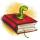Louise read from their favorite book for three days 18 pages. Every day read the same number of pages. How many pages she read every day?
2. Rolls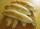Michail likes rolls, which cost 12 cents. Previously purchased 6 rolls. How many cents paid Michail for rolls?
3. Computer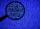A line of print on a computer contains 64 characters (letters, spacers or other chars). Find how many characters there are in 7 lines.
4. Holidays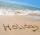Calculate how many hours take holidays, if take 5 weeks.
5. Numbers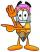By how many is the difference of numbers 8 and 34 less than its product?
6. How manzBy how many is the product of the numbers 328 and 7 greater than its sum?
7. Parking field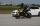There were nine cars in the parking field. The motorcycles were three times less. How many motorcycles are here and how many wheels in the parking field?
8. Car parking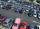The car park has 80 cars when 53 cars leaves. How many car are there?
9. Cars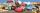Johnny has 370 cars. Peter twice less. How many cars have Peter?
10. Multiples of int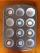How many multiples of 3 is between the numbers to 20? (zero not count)
11. The schoolThe school has 268 boys. Girls are 60 more than boys. How many children are there at school?
12. Number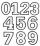Which number is 17 times larger than the number 6?
13. MultiplesFind all multiples of 10 that are larger than 136 and smaller than 214.
14. Unknown number 2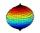I think the number. When he reduces it four times, I'll get 11. What number am I thinking?
15. Two numbersFind the four times smaller number for numbers 12 and 36 and then add them.
16. Zucchini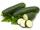One zucchini costs 5 CZK. How much would cost four?
17. Unknown number 22I am a two-digit number. My tens digits is 4. My ones digit is 3 more than my tens. Who am I?